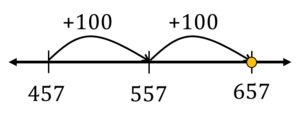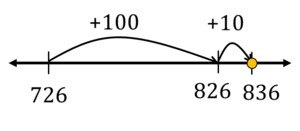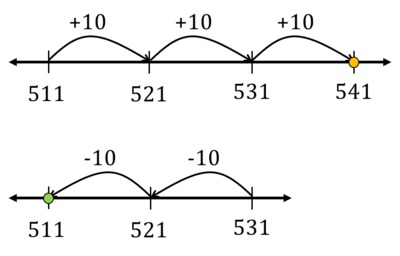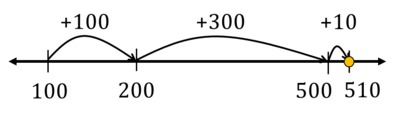# Comparisons 2

Alignments to Content Standards: 2.NBT.A.4

Use $\lt$, =, or $\gt$ to complete the following number sentences.

1. $657$ ______ $457 + 100 + 100$
2. $926$ ______ $726 + 100 +10$
3. $511 + 10 + 10 + 10$ ______ $531 - 10 - 10$
4. $923 + 10$ ______ $953 - 10 - 10$
5. $100 + 100 + 300 + 10$ ______ $510$
6. $347 + 30$ ______ $397 - 10 - 10$
7. $126 - 10 - 10 - 10 - 10$ ______ $96 - 10$

## IM Commentary

This task highlights an important part of 2.NBT.4 that previous standards often underemphasized: namely, the boldface words emphasized here:

Compare two three-digit numbers based on meanings of the hundreds, tens, and ones digits, using $\gt$, =, and $\lt$ symbols to record the results of comparisons.

The comparisons here involve sums and differences - not primarily to provide an opportunity to calculate, but rather in order to stimulate students’ thinking about the magnitudes of the base-ten units of which numbers are composed. Looking at it another way, the purpose is to provide students with an experience in using MP7, Look for and Make Use of Structure, particularly in relation to the base 10 system. Thus, for example, a student who sees the 6 hundreds in 657 and the 4 hundreds plus 2 more hundreds in 457 + 100 + 100 does not need to do the addition to see that the two expression represent the same number. Each of the parts of the problem is designed so that this sort of short cut using the structure of the base ten system is available.

In order to more fully engage students in the work of this task, the teacher may wish to have students work on the problem without paper and pencil so that they are able to reflect on the meaning of place value. In addition, the teacher may wish to model the comparisons with physical manipulatives (base ten blocks), especially if students are struggling. Understanding place value in a deep way, as required by this task, will help students develop a strong foundation for arithmetic fluency.

## Solution

1. $657 = 457+100+100$. Many approaches are possible for comparing the numbers on each side, such as:

• Both sides have a 57 and some hundreds. On the left there are 6 hundreds, and on the right there are 4 hundreds plus 2 more. So there are 6 hundreds on each side, and the two sides are equal.
• Students could notice that the tens and ones digits of the numbers on either side will be equal, and then skip-count by 100s:
Four hundred fifty-seven, five hundred fifty-seven, six hundred fifty-seven.... So the numbers on both sides are equal: 657 = 457+100+100.
• This process could also be visualized on a number line, which shows the size of the terms being added:• Of course students could also add the numbers 457, 100, and 100 vertically. That doesn’t stress thinking about the sizes of the base-ten units, however. So suppose we merely visualize doing this, rather than going to the trouble to write it down—then because of all the zeros, we can see that the tens digit of the sum will be 5 and the ones digit will be 7, while the hundreds digit will be 4 + 1 + 1 = 6. (From this one can see the relationship between the meanings of the digits, place value notation, and the vertical method.)
• $926 \gt 726+100+10$. Some possible approaches:

• On the left there are 9 hundreds, and on the right there are 7 hundreds plus 1 more hundred. So there are 9 hundreds on the left and 8 hundreds on the right. On the right there are 23+10 ones as well, which is still less than 100, so the left is greater than the right hand side.
• If the right-hand side were 726 + 100 + 100, then it would be like part (a): "726, 826, 926." But we won’t get to 926 because the last term added is much smaller than 100. So 926 $\gt$ 726 + 100 + 10.
• Showing this on a number line:• $511 + 10 + 10 + 10 \gt 531 - 10 - 10$. Some possible approaches:

• There are 5 hundreds on each side and 1 one on each side. There are 4 tens on the left and 3 minus 2 tens on the right. So the left is greater than the right hand side.

• Adding three 10's to 511: 521, 531, 541. Subtracting two 10's from 531: 521, 511. So 511 + 10 + 10 + 10 $\gt$ 531 - 10 - 10.
• Showing this on a number line:• $923 \lt 953-10-10$. Some possible approaches:

• Both sides have a 900 and a 3 and some tens. On the left there are 2 tens, and on the right there are 5 tens minus 2 tens, which is 3 tens. So the left hand side is smaller.

• Subtracting two 10's from 953: 943, 933. Adding 10 to 923 gets us to 933 as well. So 923 + 10 = 953-10-10.

• Showing this on a number line:• $100+100+300+10 = 510$. Some possible approaches:

• Both sides have 1 ten and some hundreds. On the left we have a hundred plus a hundred plus 3 hundreds, for a total of 5 hundreds. On the right there are also 5 hundreds, so the two sides are equal.

• Starting at 100, then adding another 100 gets us to 200. Adding 300 gets us to 500 since 2 hundreds plus 3 hundreds is 5 hundreds. 10 more is 510. So 100+100+300+10 = 510.

• Showing this on a number line:• $347+30 = 397-10-10$. Some possible approaches:

• Both sides have 3 hundreds and a 7 and some tens. On the left we have a 4 tens from the 347 and 3 tens from the 30, for a total of 7 tens. On the right we have a 9 tens minus 2 tens, which is also 7 tens. So the two sides are equal.

• 30 more than 347 is 377 since 4 tens plus 3 tens is 7 tens. Subtracting two 10's from 397 gets us to 387 then 377. So 347+30 = 397-10-10.

• Showing this on a number line:• $126-10-10-10-10 = 96-10$. Some possible approaches:

• Both sides have a 6. Since the left side has 1 hundred and the right side does not, it will be easier to compare the two sides if we break the hundred into 10 tens and compare the number of tens. Then on the left we have 10 tens, plus 2 more tens from the 20, minus 4 tens, for a total of 8 tens. On the right side we have 9 tens minus 1 ten, so we also have 8 tens. So the two sides are equal.

• Subtracting four 10's from 126: 116, 106, 96, 86. This is the same as subtracting one 10 from 96. So 126-10-10-10-10 = 96-10.

• Showing this on a number line: